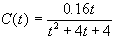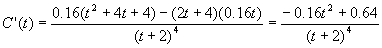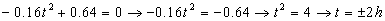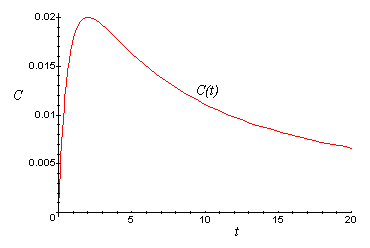# Nuclear Medicine: Determining Maximum Time for Drug Concentration

The concentration C(t) in milligrams per cubic centimeter of a particular drug in a patient’s bloodstream is given by:where t is the number of hours after the drug is taken.

1. How many hours after the drug is given will the concentration be maximum ? (Verify there is indeed a maximum at this time.)
2. What is the maximum concentration?

## Solution:

1. To find the maximum, it is necessary to find where the function for concentration has a slope or derivative = 0.

Find C′(t) using the quotient rule.Set C′(t) = 0 and solve for t:Note that time = - 2 hours does not provide a meaningful answer.

Check to see whether a maximum does occur at t = 2 hours by applying the first derivative test and checking a point on either side of C′(2).

C′(1) = +

C′(2) = 0

C′(3) = -

This means as the slopes go from + to 0 to - as t goes from 1 to 2 to 3 which maps out a maximum at time 2 h.

2. Evaluate the concentration function C(2)=0.02 mg/cc. The maximum concentration is 0.02mg/cc at time 2 hours. Here is a graph of the concentration as a function of time, verifying these results:Written by Louise Routledge, September 1, 1998